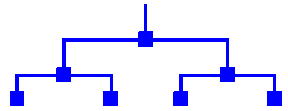binary tree

(redirected from Types of binary trees)

binary tree

[′bīn·ə·rē ′trē]
(mathematics)
A rooted tree in which each vertex has a maximum of two successors.

binary tree

(btree) A tree in which each node has at most two successors or child nodes. In Haskell this could be represented as

data BTree a = NilTree | Node a (BTree a) (BTree a)

Binary Tree